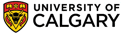Chapter 2 : AlkanesThermodynamics and Stability

• The lower the potential energy of the system, the more stable it is.
• Chemical processes usually occur because they are thermodynamically favourable (i.e. DG = -ve)
• "Thermodynamically favourable" means from high energy to low energy, or, put another way, from less stable to more stable.
• Understanding the relative stability of molecules can be important for predicting relative reactivity of starting materials and the relative yields of potential products.
• Stability can be determined by comparing experimentally measured or based on theoretical calculations.
• It is important when determining relative stabilty to compare isomeric systems.

Basics

• DG is the change in the free energy of the system.
• DH is the change in the enthalpy of the system.
• DS is the change in the entropy (i.e. disorder) of the system.
• T is the absolute temperature in Kelvin.
• R is the universal gas constant, (R = 8.314 JK-1 mol-1 or 1.986 calK-1mol-1).
• K is the equilibrium constant.

There are two very important fundamental thermodynamic equations that you should know and understand that relate various thermodynamic terms:

 DG = DH - TDS DG = -RT ln K

Heat of Reaction,  DHro

• Defined to be the heat released during a particular reaction.
• If heat is released  during this process, then the reaction is exothermic ( = heat given out)
• If heat is absorbed  during this process, then the reaction is endothermic ( = heat taken in)However, there are certain types of reactions that are particularly common for making thermodynamic comparisons:

Heat of combustion, DHco

• Defined to be the heat released when one mole of a compound undergoes complete combustion in O2.
• This will usually be an exothermic process, as shown in the example below.
• When drawing these diagrams, it is important to make sure they are balanced.
• Note that for heats of combustion, the organic compound is a starting material for the reaction.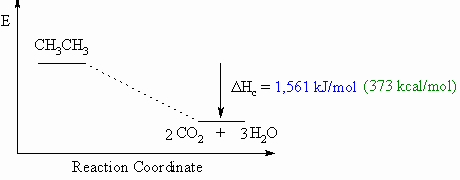Heat of formation, DHf o
• Defined to be the heat released if one mole of a compound were formed from its component elements in their standard state.
• These diagrams can be either endothermic or exothermic processes.
• When drawing these diagrams, it is important to make sure they are balanced.
• Note that for heats of formation, the organic compound is a product for the reaction.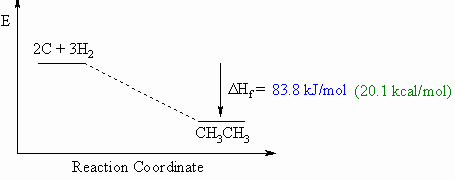Heat of hydrogenation, DHho
• Defined to be the heat released upon the addition of H2 to one mole of a compound (e.g. an alkene or alkyne) to generate the corresponding alkane.
• This will usually be an exothermic process, as shown in the example below for ethene to ethane, since 2 σ bonds are made from each π bond and each H-H σ bond.
• When drawing these diagrams, it is important to make sure they are balanced (note the inclusion of both starting materials).
• Note that for heats of hydrogenation, both starting materials and products are organic compounds.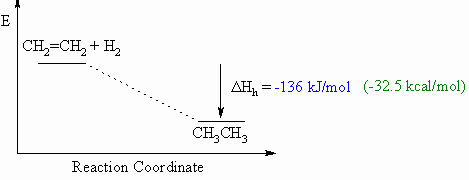Hess's Law

Although not specifically covered in most organic text books, Hess's Law is very useful when investigating the thermodynamics of reactions.  Hess's Law can be expressed by the following expression and diagram:

"The enthalpy change for a reaction (DHro) that converts starting materials to products is independent of the reaction pathway"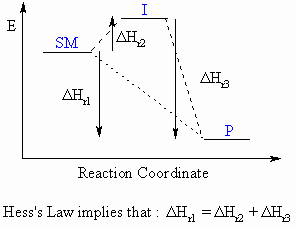For the reaction SM to P which can either proceed directly or via an intermediate, I, the overall enthalpy change for the converssion of SM to P must be the same. i.e.   DHSM-Po = DHSM-Io + DHI-Po

Essentially, it can be treated as the addition of 2-dimensional vectors, and CARE is required with the signs of the DHo terms.© Dr. Ian Hunt, Department of Chemistry